Next: D. Relation Between Charge Up: Appendix Previous: B. Expressing the Equations

# C. Estimating the Total Charge in the Diffusive Layer

The Poisson-Boltzmann equation can be solved for the total charge in the diffusive layer in a similar manner the way it is done for the semiconductor surface potential. Beginning with (5.13):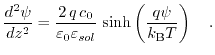(7.21)

Reexpressing it via the Debye length,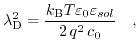(7.22)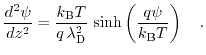(7.23)

This equation can be rewritten by applying the following identity: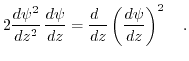(7.24)

Substituting (7.24) into (7.23) leads to a first order differential euqation: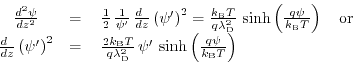(7.25)

(7.25) can be solved via separation of variables. Under the condition of a vanishing electric field for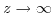the following solution can be derived: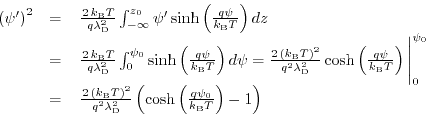(7.26)

Exploiting the identity, the expression for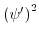can be formulated as: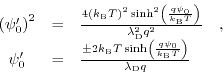(7.27)

In the last calculation step Gauß's law is utilized to express the total charge per unit area in the Gouy-Chapman layer: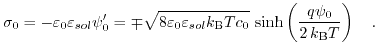(7.28)Next: D. Relation Between Charge Up: Appendix Previous: B. Expressing the Equations

T. Windbacher: Engineering Gate Stacks for Field-Effect Transistors